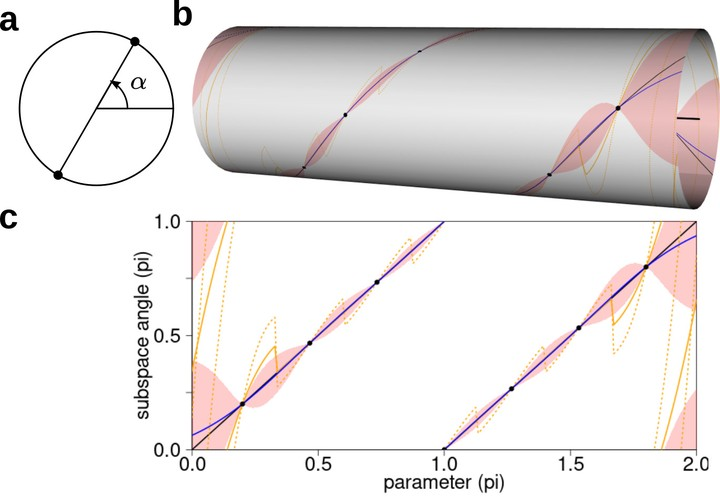# Gaussian Process Subspace Regression for Model Reduction### Abstract

Subspace-valued functions arise in a wide range of problems, including parametric reduced order modeling (PROM). In PROM, each parameter point can be associated with a subspace, which is used for Petrov-Galerkin projections of large system matrices. Previous efforts to approximate such functions use interpolations on manifolds, which can be inaccurate and slow. To tackle this, we propose a novel Bayesian nonparametric model for subspace prediction: the Gaussian Process Subspace regression (GPS) model. This method is extrinsic and intrinsic at the same time: with multivariate Gaussian distributions on the Euclidean space, it induces a joint probability model on the Grassmann manifold, the set of fixed-dimensional subspaces. The GPS adopts a simple yet general correlation structure, and a principled approach for model selection. Its predictive distribution admits an analytical form, which allows for efficient subspace prediction over the parameter space. For PROM, the GPS provides a probabilistic prediction at a new parameter point that retains the accuracy of local reduced models, at a computational complexity that does not depend on system dimension, and thus is suitable for online computation. We give four numerical examples to compare our method to subspace interpolation, as well as two methods that interpolate local reduced models. Overall, GPS is the most data efficient, more computationally efficient than subspace interpolation, and gives smooth predictions with uncertainty quantification.

Type

🏆 Winner of the INFORMS 2021 Quality, Statistics & Reliability (QSR) Best Paper Award. (First out of 27 paper submissions.)